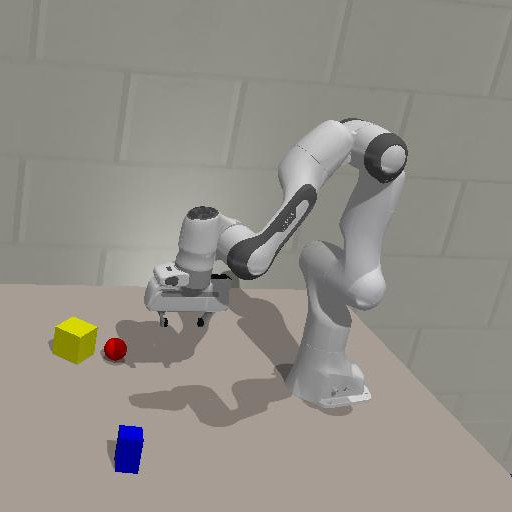Active vision

When owls scavenge for prey

Posted by Toon Van de Maele on February 19, 2021 · 6 mins read

In the case that a robots needs to manipulate, find, or do anything useful, it first needs to localize its target. Living organisms do this by first exploring the area in a seemingly intelligent manner, choosing locations they have not yet visited. When we are searching for something and find a cue that could potentially lead to the solution, we, like any other organism, will investigate this further to evaluate whether this leads to our target. As this is a logical approach to searching, we ask the question: “Can we mimic this exploratory behaviour by driving viewpoint selection through the active inference paradigm?”

We try to answer this question using a simulated robot manipulator in a workspace with blocks of varying shapes and colors in which the robot is tasked to find a single object.

Expected Free Energy

Active inference posits that living agents minimize their free energy by choosing specific actions. As it is impossible to know what will happen in the future, we can only estimate the free energy at a future point by computing the expected free energy G with respect to a potential viewpoint to visit.

$\begin{equation} \begin{split} G(\mathbf{v}_{i+1}) &\approx -\underbrace{ \mathbb{E}_{Q(\mathbf{o}_{i+1}| \mathbf{o}_{0:i},\mathbf{v}_{0:i+1})}\big[ \text{D}_\text{KL}[Q(\mathbf{s}|\mathbf{o}_{0:i+1}, \mathbf{v}_{0:i+1})||Q(\mathbf{s}|\mathbf{o}_{0:i}, \mathbf{v}_{0:i})]\big]}_{\text{Epistemic value}} \\ &\quad- \underbrace{\mathbb{E}_{Q(\mathbf{o}_{i+1}| \mathbf{o}_{0:i},\mathbf{v}_{0:i+1})}[ \log P(\mathbf{o}_{0:i+1})]}_{\text{Instrumental value}} \label{eq:efe} \end{split} \end{equation}$

Intuitively, this equation can be dissected in an epistemic and an instrumental term. The epistemic term computes how much the belief over the environment will change when visiting this novel viewpoint, and thus is a quantification of how much the robot can learn by moving to this position. The instrumental term, on the other hand, reflects how close the expected observation is to the target view the agent is trying to reach.

Generative Model

From the equation of G, it is clear that two elements are crucial in computing the expected free energy: a belief over the environment and the ability to imagine what will be observed. Similar to the blog post on robot imaginations, we learn a generative model by minimizing its surprise from prerecorded sequences to do just that.

As we consider the world to be unchanging, the model is slightly different from the previous post. The generative model consists of a posterior model that estimates the belief $$p(\mathbf{s} \vert \mathbf{o})$$ over the environment from a sequence of observations, and a likelihood model that estimates the most likely observation to encounter when visiting a potential viewpoint. In the video below, you can see imaginative movement of the robotic gripper after scanning the scene shown on the right.

 Your browser does not support the video tag.Also, the model will integrate information as new views are collected. For example in the next video, you see how various viewpoints are imagined as more observations are gathered. Before the initial observation, the robot has no idea about the scene and will imagine random objects. After the first observation, the model roughly encodes the location of one blue and two yellow objects. The more observations are integrated, the better the model encodes the position and shape of the different objects in the scene.

Emerging behaviour

When we limit the robotic agent in height, we observe exploring behavior that scans the environment until it has found the target cube. This target is provided to the agent only as an observation. For example in the video below, the robot first moves to a different part of the scene to find yellow objects. Once these are spotted, it can infer the target pose, and moves to that particular location.

In a second experiment, we lift this limitation and allow the robotic agent to move in all three degrees of freedom. Not only does the agent reach its target faster than before, it also first moves upwards to acquire a broader scan of the environment, similar to how owls move to a high vantage point to scavenge for their prey.

Potential

The results for this paper show that we can create an intelligent robot that is able to search a real workspace using a learnt generative model using a generic objective. The fact that this objective works using only the imagined transition of belief over the environment and its corresponding observations after selecting a new viewpoint or action show that this approach could easily be extended to more complex use cases with a different generative model. If this model is complex and accurate enough to properly predict the outcome for a large set of possible actions, it could, for example, do manipulation tasks when provided with only a view of the desired solution.

For specifics on how to compute the expected free energy, the neural network parameters and other technical details, we refer you to the scientific paper.